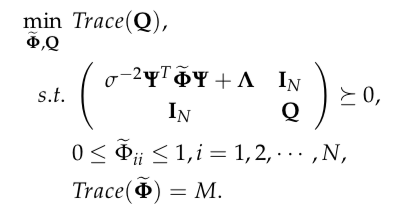A semidefinite programming problem

(gary) #1

Hello,

I try to implement the problem as follows. Φ,Q are my variables and both are NxN diagonal matricesMy Matlab code is shown as below:However, the result is not as expected. I think maybe something wrong in my code.
Can anyone tell me that my code is correct or not? Thank you!

(Mark L. Stone) #2

In the future, please copy and paste code into your post and use the Preformatted text icon. Do not post images of your code.

You should have declared
variable Q(n,n) diagonal
if Q is supposed to be diagonal, as you say.

What is I? If t has not already been set to eye(n), the code will not be correct.

Presumably, initsiigma = \sigma^{-2}

A for loop can be avoided by using
0 <= diag(Phi) <= 1
or
0 <= Phi(:) <= 1
although your implementation of that constraint is not wrong.

1 Like